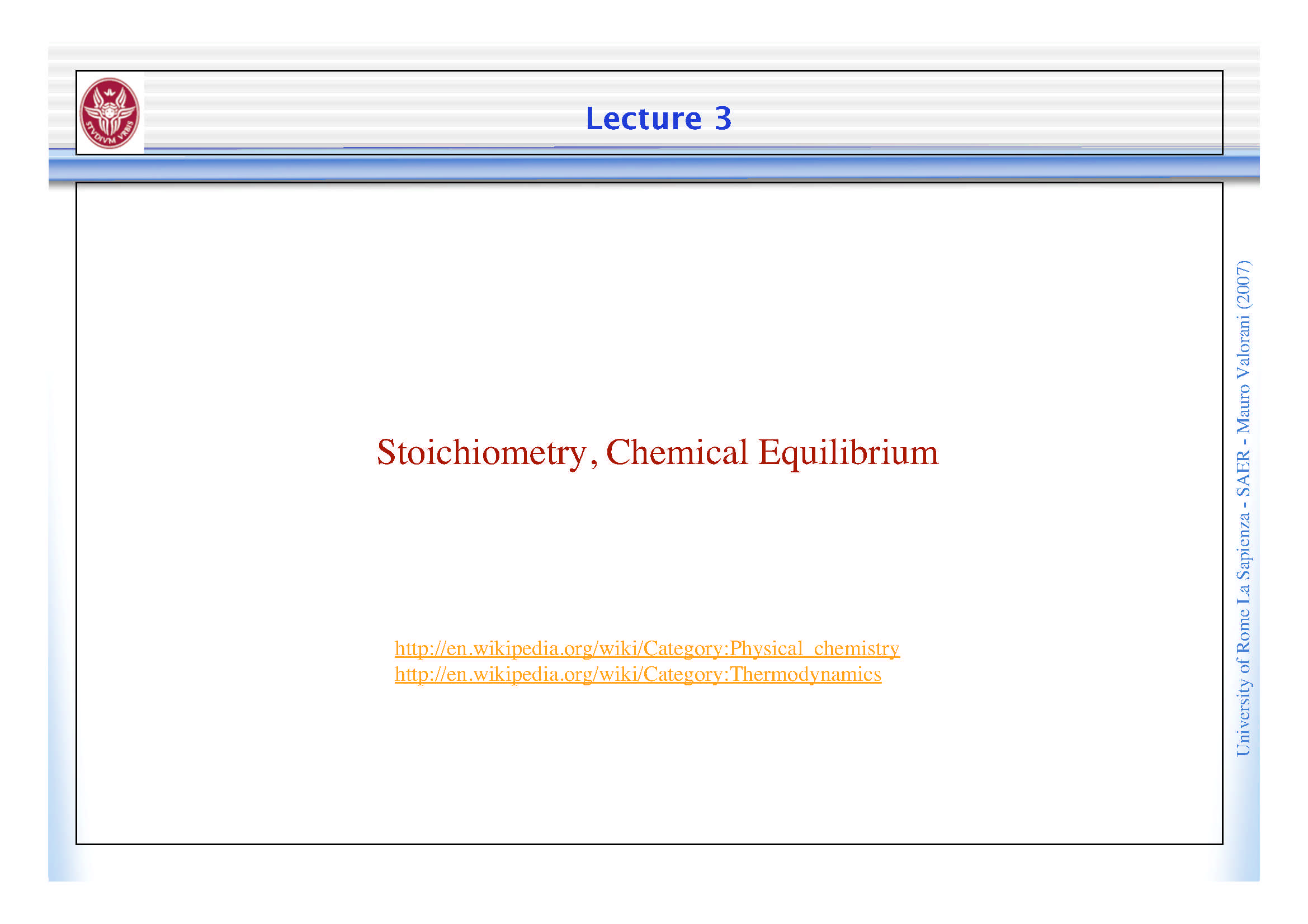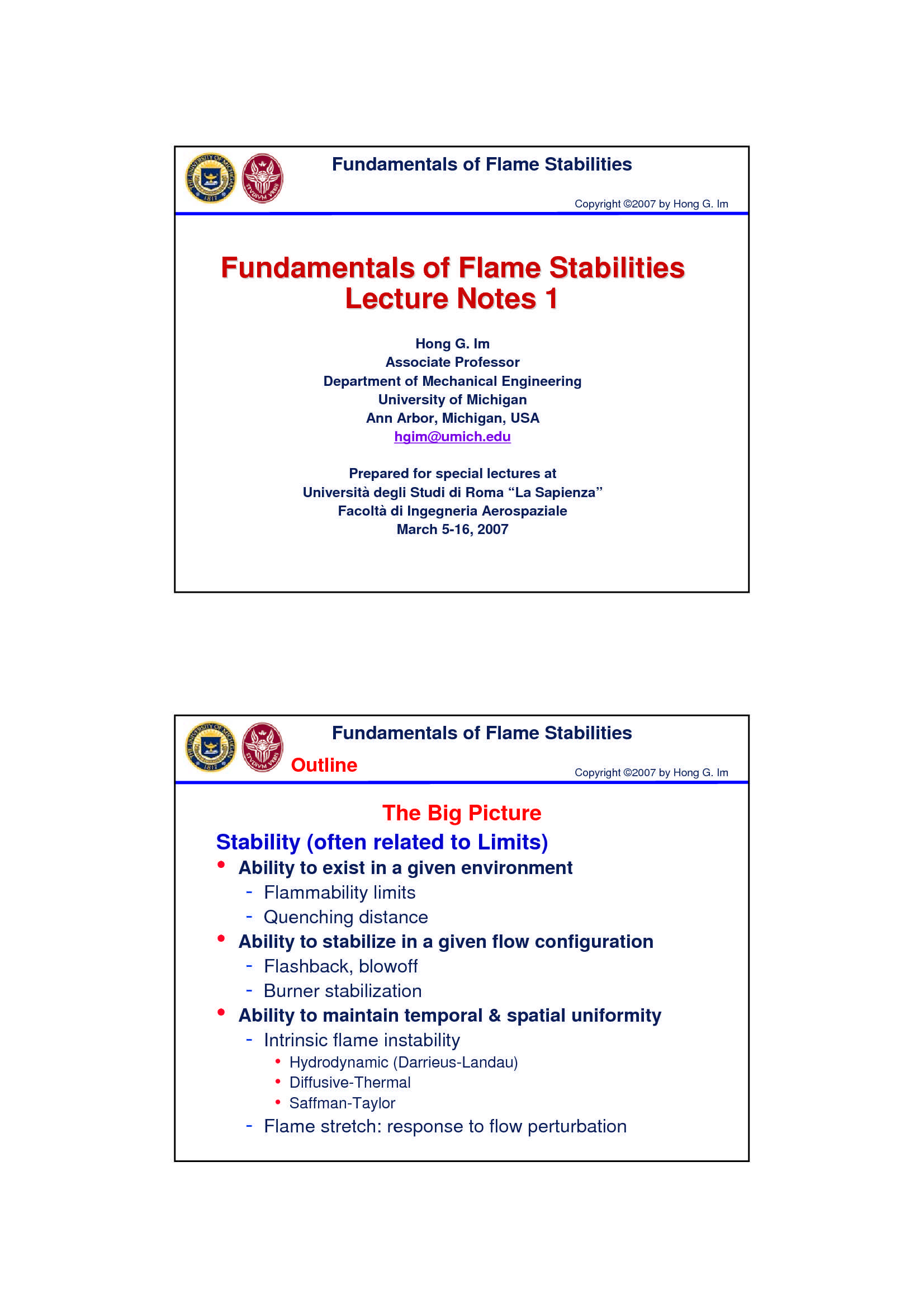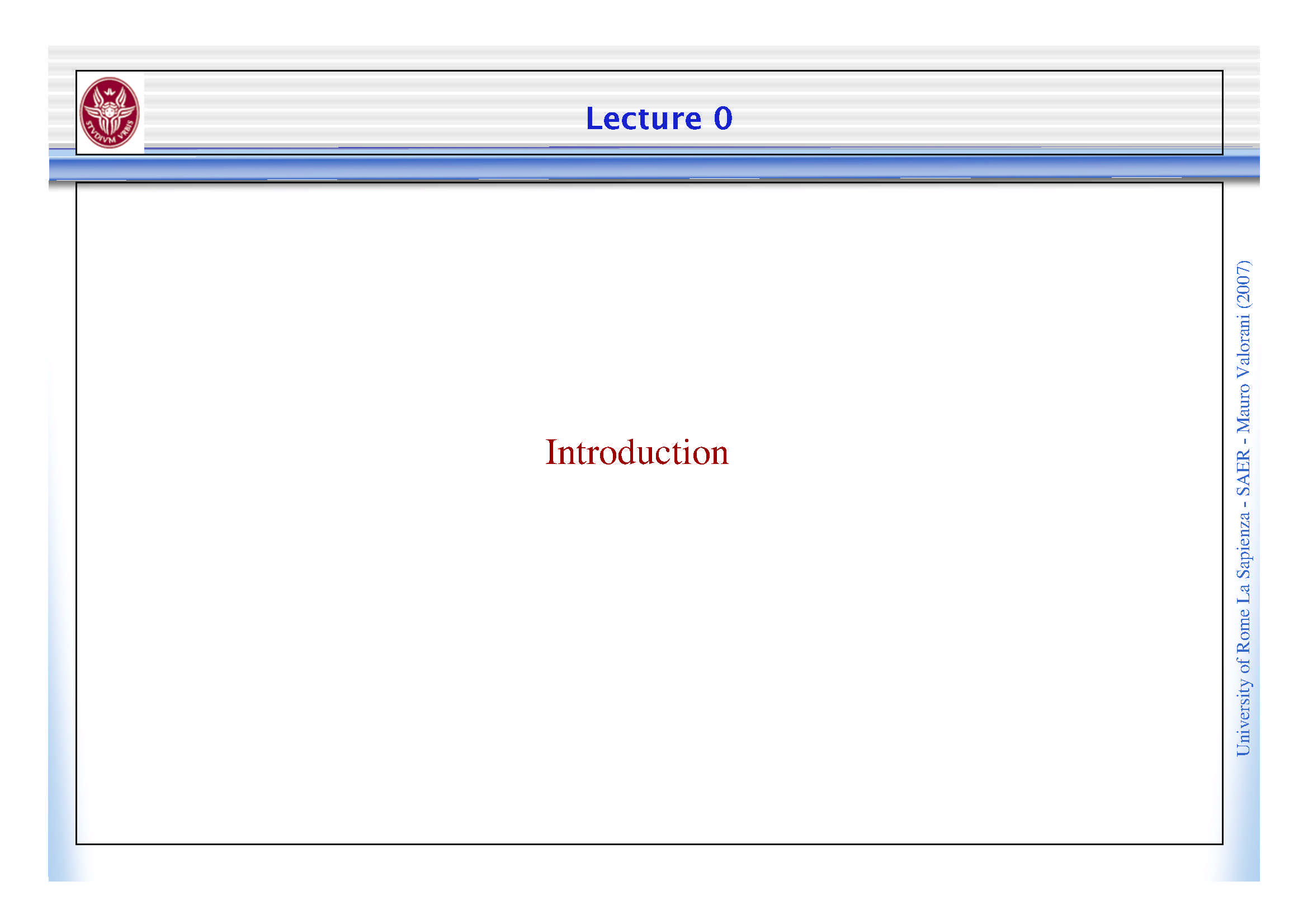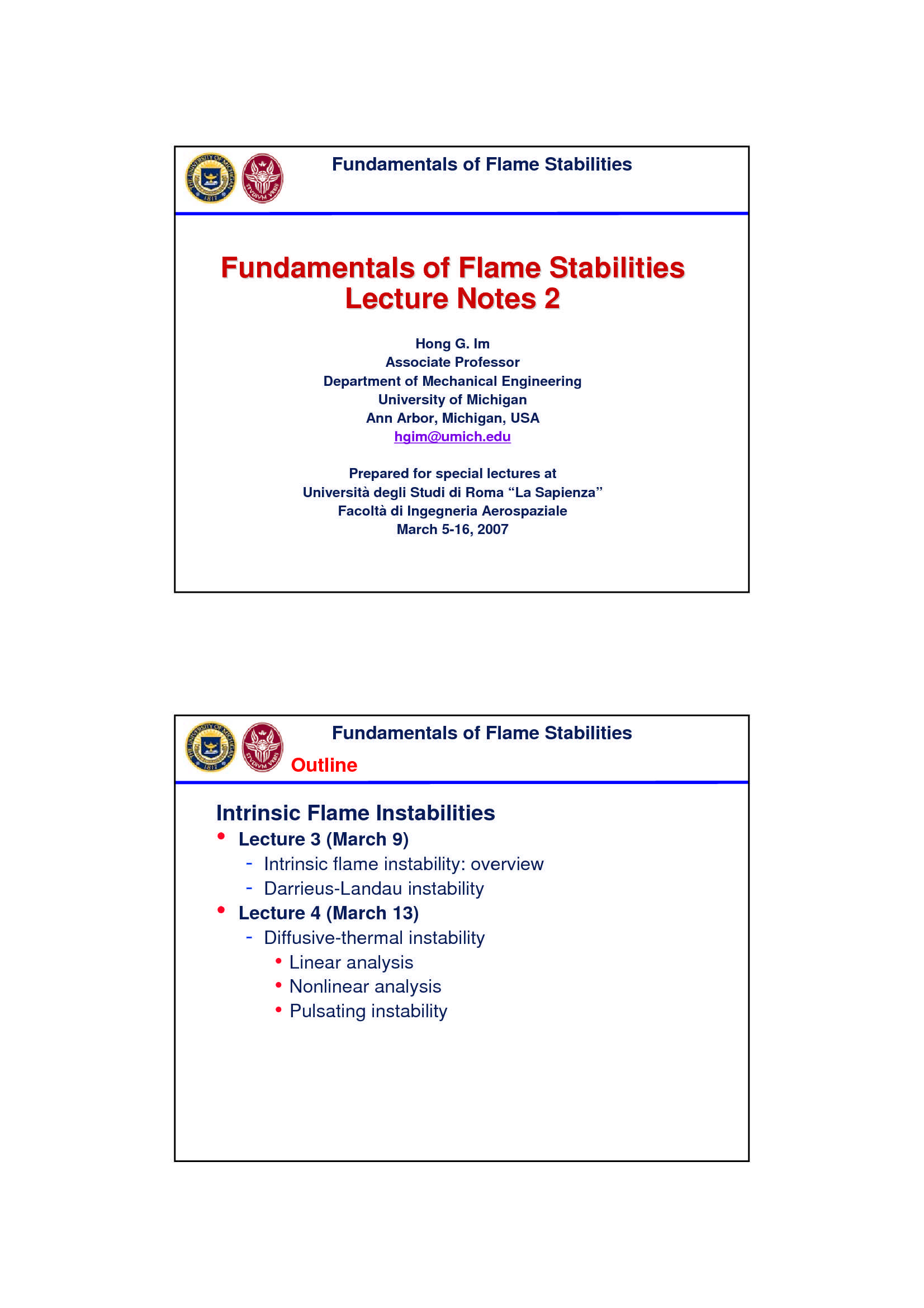Che materia stai cercando?

# Termodinamica del non equilibrio

Materiale didattico per il corso di Combustione del Prof. Mauro Valorani, all'interno del quale sono affrontati i seguenti argomenti: peso atomico e peso molecolare; composizione di miscele gassose; principi di termodinamica del non equilibrio; potenziali termodinamici ed energia libera di Helmoltz; energia... Vedi di più

Esame di Combustione docente Prof. M. Valorani

Anteprima

### ESTRATTO DOCUMENTO

Molecular Weight (2007)

= Valorani

j 1 N

j j

a a

1 NE Mauro

-

Natoms

! SAER

=

j ij i

W a W -

=1 Sapienza

i La

" = # + # + #

## CH O C H O

CH O W 1 W 3 W 1 W

3 Rome

3 = # + # + # =

1 12 3 1 1 16 31 of

University

Extensive and Intensive Properties (2007)

! Valorani

3

! ! Mauro

4 2 -

## SAER

! -

1 Sapienza

"

! !

=

Extensive Property: La

j Rome

=1,

j N

! =

Intensive Property: const j=1,N of

j University

Composition of a Gaseous Mixture

Extensive Variables: (2007)

4 4

" "

! = =

j j

Number of Particles is extensive N (N ) ; N (N )

part ,i part ,i part part

Number of Particles N [ part] =1 =1

part ,i j j Valorani

Nspecies 4 4

" "

" ! = =

! = j j

Mole is extensive N (N ) ; N (N )

Mole N [mol] N N i i

i i =1 =1

=1 j j

i

Nspecies 4 4

" " " Mauro

! = ! = =

j j

Mass M [Kg] M M Mass is extensive M (M ) ; M (M )

i i i i

=1 =1 =1

i j j -

## SAER

Intensive Variables:

N part

= part ,i

Number Density n [ ] -

( ) ( )

i 3 Sapienza

V m 1 4

!

Number Densities are intensive n =…= n

part ,i part ,i

Nspecies [ ]

!

[ ] = ( ) ( )

n n 1 4

i n =…= n

=1 part part

i La

( ) ( )

[ ] [ ]

N mol

[ ] 1 4

!

= Rome

Molar Concentrations are intensive N =...= N

i

Molar Concentration N [ ] i i

i 3

V m ( ) ( )

[ ] [ ]

1 4

N =...= N

Nspecies [ ] of

!

[ ] =

N N ( ) ( ) University

i 1 4

" "

!

Densities are intensive = ... =

=1

i i i

( ) ( )

M Kg " "

1 4

" = = ... =

i

Partial Density [ ]

i 3

V m

Nspecies

!

" "

= i

=1

i Fuel/Oxidant Parameters

! ! !

= + "

For any extensive property: f (1 f ) (2007)

mix F OX Valorani

! !

"

= mix OX

Mixture Fraction f Mauro

! !

"

F OX -

## SAER

"

o 1 f -

Sapienza

=

Mixture Ratio f f La

Rome

$' f of & ) University % ( o # = Equivalence Ratio$ '

f

& )

% (

o stechio

Mass and Molar Fractions

Definition [ ] (2007)

## N

= = i

i

Molar Fraction X [!]

[ ] Valorani

i N N

"

M Mauro

= i i

Mass Fraction Y = [!]

"

i M -

## SAER

Transformation Rules -

Sapienza

=

i i i La

=

M WN Rome

of

University

= i i i i

Y = = X

i i

## M WN W

Principles of Nonequilibrium Thermodynamics (2007)

Assumptions: Valorani

System in local thermal and mechanical equilibrium

System in local chemical nonequilibrium Mauro

-

State variables: SAER

Pressure (intensive) p -

Sapienza

Volume (extensive) V

Moles (extensive) N i La

Rome

of

University

Zero-th Principle of Nonequilibrium Thermodynamics

There exists an intensive variable, the temperature T, which is a state function

obeying to the equation of state: (2007)

=

T T ( p,V, N ) Valorani

j Mauro

T is the same for systems A, B, C if they are in thermodynamic equilibium, that is: -

= SAER

If T T

= -

and T T Sapienza

## B C

=

then T T La

A B Rome

The Zero-th Principle ensures the existence of a Thermal Equation of State of the of

form: University

=

p p(T ,V, N )

j

First Principle of Nonequilibrium Thermodynamics

There exists an extensive variable, the internal energy U, which is a state (2007)

function U=U(p,V,N ), and whose variation can be computed as:

j Valorani

Mauro

Nspecies

#

! ! µ

= " +

dU Q W dN

Open system -

j j SAER

=1

j -

Sapienza

! !

= "

dU Q W La

Closed system (dN =0) Rome

j of

University

First Principle: Sensible Energy

The internal energy U is a measure of the energy stored in the internal degrees (2007)

of freedom of the molecule: Valorani

Translational energy Mauro

Rotational energy

Vibrational energy -

## SAER

-

These contribution can be computed by the Partition Functions obtained via Sapienza

Statistical Mechanics La

Rome

Under condition of thermal equilibrium, these contributions depends only on of

temperature, and for this reason, they form the sensible energy University

First Principle: Energy of Formation

Since all processes at the molecular level are conservative, then the internal (2007)

energy of a gas is an invariant and only variation of internal energy are of

interest; Valorani

Thus, a reference level is required to define defined U, named energy of Mauro

formation : -

## SAER

1) The energy of formation of pure substances at standard conditions is 0 by -

Sapienza

definition;

2) The energy of formation of a compound is found by adding the bounding La

energy needed to form it from pure substances. Rome

of

University

Second Principle of Nonequilibrium Thermodynamics

There exists:

1) an absolute scale of the temperature, and (2007)

2) an extensive variable, the entropy S, which is a state function S=S(p,V,N ),

j Valorani

whose variation for a closed system can be computed as:

"

! Mauro

TdS Q -

where the sign : SAER

= holds for changes due to reversible processes -

Sapienza

> holds for changes due to irreversible processes

For a systems with both external and internal irreversible processes: La

Rome

= +

dS d S d S of

ext int University

=

dS d S interaction with the outside of the system

ext

=

dS d S due to irreversible processes inside the system

int

PAGINE

26

PESO

2.04 MB

AUTORE

PUBBLICATO

+1 anno fa

### DESCRIZIONE DISPENSA

Materiale didattico per il corso di Combustione del Prof. Mauro Valorani, all'interno del quale sono affrontati i seguenti argomenti: peso atomico e peso molecolare; composizione di miscele gassose; principi di termodinamica del non equilibrio; potenziali termodinamici ed energia libera di Helmoltz; energia libera di Gibbs.

DETTAGLI
Esame: Combustione
Corso di laurea: Corso di laurea magistrale in ingegneria aeronautica
SSD:
A.A.: 2007-2008

I contenuti di questa pagina costituiscono rielaborazioni personali del Publisher Atreyu di informazioni apprese con la frequenza delle lezioni di Combustione e studio autonomo di eventuali libri di riferimento in preparazione dell'esame finale o della tesi. Non devono intendersi come materiale ufficiale dell'università La Sapienza - Uniroma1 o del prof Valorani Mauro.

Acquista con carta o conto PayPal

Scarica il file tutte le volte che vuoi

Paga con un conto PayPal per usufruire della garanzia Soddisfatto o rimborsato

RecensioniTi è piaciuto questo appunto? Valutalo!

## Altri appunti di CombustioneDispensa

### Stechiometria ed equilibrio chimicoDispensa

### Stabilità della fiammaDispensa

### Combustione omogenea e combustione eterogeneaDispensa